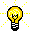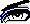#Math on Wheels### Learning by Problem Solving:Suppose we want to write a program in Maple for visualizaing the Pascal's triangle. The MathResource interactive Dictionary of Mathematics [by J.M. Borwein, C.R.Watters and E.J. Borowski] defines the Pascal's triangle as follows:

Pascal's triangle, n. the triangular array of integers, with 1 at the apex, in which each number is the sum of the two numbers above it in the preceding row; an initial segment is shown in Fig. 279 [ reproduced below ]. The nth line of the triangle is the sequence of coefficients of x^{k}a^{n-k} in the expansion of the binomial (x + a)^{n}.

```

1
1     1
1     2     1
1     3     3     1
1     4     6     4     1
1  .........................  1

```Studying the pattern of even and odd numbers in the triangle provides a basis and motivation for visualization: for now, lets choose to display even numbers in the triangle using red dots and the odd numbers using blue dots. Noting that each element of the rows of the triangle is just the binomial coefficients n choose k as k runs from 0 to n, we can write Maple code that computes the elements of Pascal's triangle:

1. using the built-in binomial command, generate the rows of the triangle
2. decide on which colors to assign to even and odd elements,
3. and finally, produce a PLOT data structure, which is then displayed or printed on some specified plotdevice.Here is the Maple code (courtesy of Michael Monagan) that implements the above visualization scheme:

```

N := 63;

binrow := proc(n) local i,r,c,j;
for j from 0 to n do
for i from 0 to j do
if binomial(j,i) mod 2 = 1 then c := 0,0,1 else c := 1,0,0 fi;
r[j,i] := POINTS([i+(n-j)/2, n-j], SYMBOL(CIRCLE), COLOR(RGB,c))
od:
od:
PLOT( seq(seq(r[j,i],i=0..j),j=0..n), AXESSTYLE(NONE) )
end;

binrow(N);

```After clicking on the maple leaf icon to the left , experiment with the above code by increasing the number of rows from 63 to 85 in the Maple Form Interface. Simply edit the first line of the program in the input form window, and change N := 63 to N := 85.
This help page, the Maple Form Interface, and the Annotation Form Interface were created and are maintaind by David Fayegh (fayegh@cecm.sfu.ca)